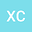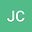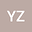Differentiability on time and continuity on fractional order of solutions for Caputo fractional evolution equations
•••• Xingfa Chen,
• Junfei Cao,
• Yong Zhou
Xingfa Chen
Guangdong University of Education

Corresponding Author:chenxingfa@gdei.edu.cn

Author ProfileJunfei Cao
Guangdong University of Education
Author ProfileYong Zhou
Xiangtan University
Author Profile## Abstract

In this paper, the differentiability on time and continuity on fractional order of solutions for a class of Caputo fractional evolution equations are studied. Under appropriate assumptions, the existence and differentiability on time of solutions for linear as well as semilinear Caputo fractional evolution equations are analyzed, the continuity of solutions on fractional order for linear and semilinear Caputo fractional evolution equations are discussed. In addition, if the fractional order converges to $1$, then the solutions of the Caputo fractional differential equations become the solutions of classic evolution equations. The continuity of solutions on fractional order for some fractional systems is numerically studied, and the results are basically consistent with the theoretical results.